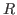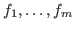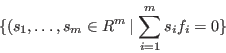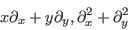: Control Structures and programming : Gröbner basis and Syzygy : Computing Gröbner basis in   目次

## Computing syzygies

Letbe a ring andbe elements of. The left-moduleis called the syzygy among. The following script computes the generators of the syzygy amongin the homogenized Weyl algebra.

%% syz.sm1

[ (x,y) ring_of_differential_operators
[[(Dx) 1 (Dy) 1]] weight_vector
0
] define_ring

[ (x Dx + y Dy).
(Dx^2 + Dy^2).
] /ff set

ff { [[(h). (1).]] replace homogenize} map /ff2 set

[ff2 [(needBack) (needSyz)]] groebner /ans set ;
(Syzygies are ...) message
ans 2 get ::

The 0-th element of ans is the Gröbner basis. The 1st element of ans is the transformation matrix from the input to the Gröbner basis. The 2nd element of ans is a set of generators of the syzygies of the input.

Nobuki Takayama 平成22年2月8日